# Modeling Correlated Defaults with Copulas

This example explores how to simulate correlated counterparty defaults using a multifactor copula model.

Potential losses are estimated for a portfolio of counterparties, given their exposure at default, default probability, and loss given default information. A `creditDefaultCopula` object is used to model each obligor's credit worthiness with latent variables. Latent variables are composed of a series of weighted underlying credit factors, as well as, each obligor's idiosyncratic credit factor. The latent variables are mapped to an obligor's default or nondefault state for each scenario based on their probability of default. Portfolio risk measures, risk contributions at a counterparty level, and simulation convergence information are supported in the `creditDefaultCopula` object.

This example also explores the sensitivity of the risk measures to the type of copula (Gaussian copula versus t copula) used for the simulation.

### Load and Examine Portfolio Data

The portfolio contains 100 counterparties and their associated credit exposures at default (`EAD`), probability of default (`PD`), and loss given default (`LGD`). Using a `creditDefaultCopula` object, you can simulate defaults and losses over some fixed time period (for example, one year). The `EAD`, `PD`, and `LGD` inputs must be specific to a particular time horizon.

In this example, each counterparty is mapped onto two underlying credit factors with a set of weights. The `Weights2F` variable is a `NumCounterparties-by-3` matrix, where each row contains the weights for a single counterparty. The first two columns are the weights for the two credit factors and the last column is the idiosyncratic weights for each counterparty. A correlation matrix for the two underlying factors is also provided in this example (`FactorCorr2F`).

```load CreditPortfolioData.mat whos EAD PD LGD Weights2F FactorCorr2F ```
``` Name Size Bytes Class Attributes EAD 100x1 800 double FactorCorr2F 2x2 32 double LGD 100x1 800 double PD 100x1 800 double Weights2F 100x3 2400 double ```

Initialize the `creditDefaultCopula` object with the portfolio information and the factor correlation.

```rng('default'); cc = creditDefaultCopula(EAD,PD,LGD,Weights2F,'FactorCorrelation',FactorCorr2F); % Change the VaR level to 99%. cc.VaRLevel = 0.99; disp(cc) ```
``` creditDefaultCopula with properties: Portfolio: [100x5 table] FactorCorrelation: [2x2 double] VaRLevel: 0.9900 UseParallel: 0 PortfolioLosses: [] ```
```cc.Portfolio(1:5,:) ```
```ans = 5x5 table ID EAD PD LGD Weights __ ______ _________ ____ ____________________ 1 21.627 0.0050092 0.35 0.35 0 0.65 2 3.2595 0.060185 0.35 0 0.45 0.55 3 20.391 0.11015 0.55 0.15 0 0.85 4 3.7534 0.0020125 0.35 0.25 0 0.75 5 5.7193 0.060185 0.35 0.35 0 0.65 ```

### Simulate the Model and Plot Potential Losses

Simulate the multifactor model using the `simulate` function. By default, a Gaussian copula is used. This function internally maps realized latent variables to default states and computes the corresponding losses. After the simulation, the `creditDefaultCopula` object populates the `PortfolioLosses` and `CounterpartyLosses` properties with the simulation results.

```cc = simulate(cc,1e5); disp(cc) ```
``` creditDefaultCopula with properties: Portfolio: [100x5 table] FactorCorrelation: [2x2 double] VaRLevel: 0.9900 UseParallel: 0 PortfolioLosses: [1x100000 double] ```

The `portfolioRisk` function returns risk measures for the total portfolio loss distribution, and optionally, their respective confidence intervals. The value-at-risk (VaR) and conditional value-at-risk (CVaR) are reported at the level set in the `VaRLevel` property for the `creditDefaultCopula` object.

```[pr,pr_ci] = portfolioRisk(cc); fprintf('Portfolio risk measures:\n'); disp(pr) fprintf('\n\nConfidence intervals for the risk measures:\n'); disp(pr_ci) ```
```Portfolio risk measures: EL Std VaR CVaR ______ ______ _____ ______ 24.876 23.778 102.4 121.28 Confidence intervals for the risk measures: EL Std VaR CVaR ________________ ________________ ________________ ________________ 24.729 25.023 23.674 23.883 101.19 103.5 120.13 122.42 ```

Look at the distribution of portfolio losses. The expected loss (EL), VaR, and CVaR are marked as the vertical lines. The economic capital, given by the difference between the VaR and the EL, is shown as the shaded area between the EL and the VaR.

```histogram(cc.PortfolioLosses) title('Portfolio Losses'); xlabel('Losses (\$)') ylabel('Frequency') hold on % Overlay the risk measures on the histogram. xlim([0 1.1 * pr.CVaR]) plotline = @(x,color) plot([x x],ylim,'LineWidth',2,'Color',color); plotline(pr.EL,'b'); plotline(pr.VaR,'r'); cvarline = plotline(pr.CVaR,'m'); % Shade the areas of expected loss and economic capital. plotband = @(x,color) patch([x fliplr(x)],[0 0 repmat(max(ylim),1,2)],... color,'FaceAlpha',0.15); elband = plotband([0 pr.EL],'blue'); ulband = plotband([pr.EL pr.VaR],'red'); legend([elband,ulband,cvarline],... {'Expected Loss','Economic Capital','CVaR (99%)'},... 'Location','north'); ```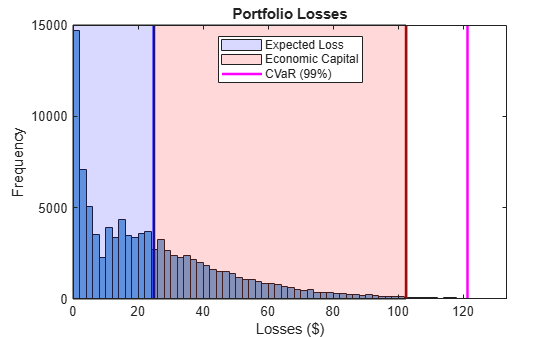### Find Concentration Risk for Counterparties

Find the concentration risk in the portfolio using the `riskContribution` function. `riskContribution` returns the contribution of each counterparty to the portfolio EL and CVaR. These additive contributions sum to the corresponding total portfolio risk measure.

```rc = riskContribution(cc); % Risk contributions are reported for EL and CVaR. rc(1:5,:) ```
```ans = 5x5 table ID EL Std VaR CVaR __ ________ __________ _________ _________ 1 0.036031 0.022762 0.083828 0.13625 2 0.068357 0.039295 0.23373 0.24984 3 1.2228 0.60699 2.3184 2.3775 4 0.002877 0.00079014 0.0024248 0.0013137 5 0.12127 0.037144 0.18474 0.24622 ```

Find the riskiest counterparties by their CVaR contributions.

```[rc_sorted,idx] = sortrows(rc,'CVaR','descend'); rc_sorted(1:5,:) ```
```ans = 5x5 table ID EL Std VaR CVaR __ _______ ______ ______ ______ 89 2.2647 2.2063 8.2676 8.9997 96 1.3515 1.6514 6.6157 7.7062 66 0.90459 1.474 6.4168 7.5149 22 1.5745 1.8663 6.0121 7.3814 16 1.6352 1.5288 6.3404 7.3462 ```

Plot the counterparty exposures and CVaR contributions. The counterparties with the highest CVaR contributions are plotted in red and orange.

```figure; pointSize = 50; colorVector = rc_sorted.CVaR; scatter(cc.Portfolio(idx,:).EAD, rc_sorted.CVaR,... pointSize,colorVector,'filled') colormap('jet') title('CVaR Contribution vs. Exposure') xlabel('Exposure') ylabel('CVaR Contribution') grid on ```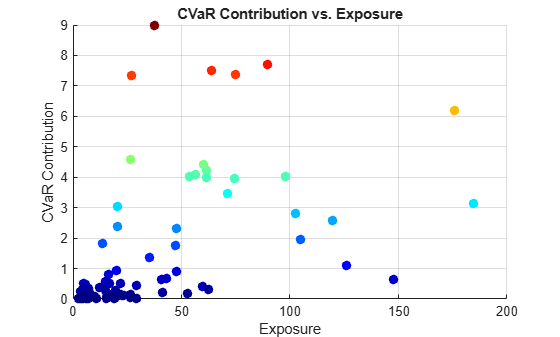### Investigate Simulation Convergence with Confidence Bands

Use the `confidenceBands` function to investigate the convergence of the simulation. By default, the CVaR confidence bands are reported, but confidence bands for all risk measures are supported using the optional `RiskMeasure` argument.

```cb = confidenceBands(cc); % The confidence bands are stored in a table. cb(1:5,:) ```
```ans = 5x4 table NumScenarios Lower CVaR Upper ____________ ______ ______ ______ 1000 106.7 121.99 137.28 2000 109.18 117.28 125.38 3000 114.68 121.63 128.58 4000 114.02 120.06 126.11 5000 114.77 120.36 125.94 ```

Plot the confidence bands to see how quickly the estimates converge.

```figure; plot(... cb.NumScenarios,... cb{:,{'Upper' 'CVaR' 'Lower'}},... 'LineWidth',2); title('CVaR: 95% Confidence Interval vs. # of Scenarios'); xlabel('# of Scenarios'); ylabel('CVaR + 95% CI') legend('Upper Band','CVaR','Lower Band'); grid on ```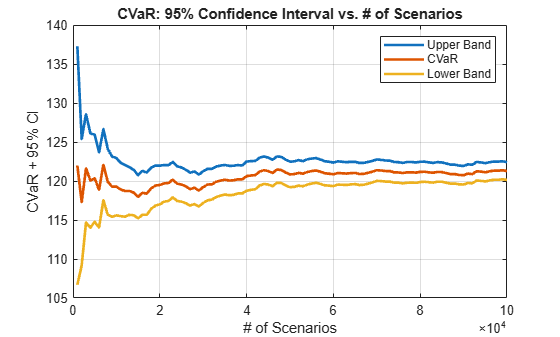Find the necessary number of scenarios to achieve a particular width of the confidence bands.

```width = (cb.Upper - cb.Lower) ./ cb.CVaR; figure; plot(cb.NumScenarios,width * 100,'LineWidth',2); title('CVaR: 95% Confidence Interval Width vs. # of Scenarios'); xlabel('# of Scenarios'); ylabel('Width of CI as %ile of Value') grid on % Find point at which the confidence bands are within 1% (two sided) of the % CVaR. thresh = 0.02; scenIdx = find(width <= thresh,1,'first'); scenValue = cb.NumScenarios(scenIdx); widthValue = width(scenIdx); hold on plot(xlim,100 * [widthValue widthValue],... [scenValue scenValue], ylim,... 'LineWidth',2); title('Scenarios Required for Confidence Interval with 2% Width'); ```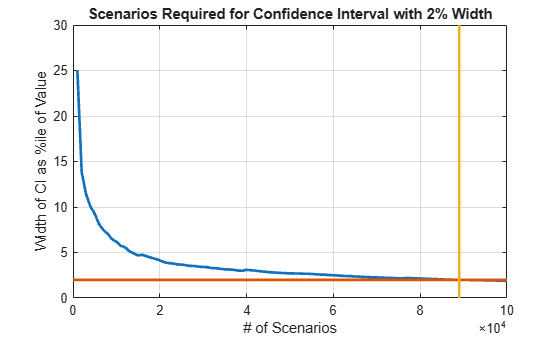### Compare Tail Risk for Gaussian and t Copulas

Switching to a t copula increases the default correlation between counterparties. This results in a fatter tail distribution of portfolio losses, and in higher potential losses in stressed scenarios.

Rerun the simulation using a t copula and compute the new portfolio risk measures. The default degrees of freedom (dof) for the t copula is five.

```cc_t = simulate(cc,1e5,'Copula','t'); pr_t = portfolioRisk(cc_t); ```

See how the portfolio risk changes with the t copula.

```fprintf('Portfolio risk with Gaussian copula:\n'); disp(pr) fprintf('\n\nPortfolio risk with t copula (dof = 5):\n'); disp(pr_t) ```
```Portfolio risk with Gaussian copula: EL Std VaR CVaR ______ ______ _____ ______ 24.876 23.778 102.4 121.28 Portfolio risk with t copula (dof = 5): EL Std VaR CVaR ______ ______ ______ ______ 24.808 38.749 186.08 250.59 ```

Compare the tail losses of each model.

```% Plot the Gaussian copula tail. figure; subplot(2,1,1) p1 = histogram(cc.PortfolioLosses); hold on plotline(pr.VaR,[1 0.5 0.5]) plotline(pr.CVaR,[1 0 0]) xlim([0.8 * pr.VaR 1.2 * pr_t.CVaR]); ylim([0 1000]); grid on legend('Loss Distribution','VaR','CVaR') title('Portfolio Losses with Gaussian Copula'); xlabel('Losses (\$)'); ylabel('Frequency'); % Plot the t copula tail. subplot(2,1,2) p2 = histogram(cc_t.PortfolioLosses); hold on plotline(pr_t.VaR,[1 0.5 0.5]) plotline(pr_t.CVaR,[1 0 0]) xlim([0.8 * pr.VaR 1.2 * pr_t.CVaR]); ylim([0 1000]); grid on legend('Loss Distribution','VaR','CVaR'); title('Portfolio Losses with t Copula (dof = 5)'); xlabel('Losses (\$)'); ylabel('Frequency'); ```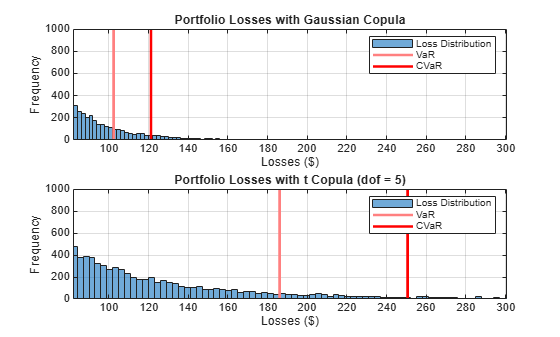The tail risk measures VaR and CVaR are significantly higher using the t copula with five degrees of freedom. The default correlations are higher with t copulas, therefore there are more scenarios where multiple counterparties default. The number of degrees of freedom plays a significant role. For very high degrees of freedom, the results with the t copula are similar to the results with the Gaussian copula. Five is a very low number of degrees of freedom and, consequentially, the results show striking differences. Furthermore, these results highlight that the potential for extreme losses are very sensitive to the choice of copula and the number of degrees of freedom.

﻿